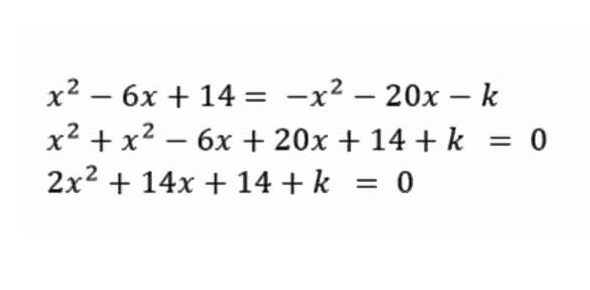Which of the following lines does not intersect y = 5 x + 2? - ProProfs DiscussTopicsMoreProductsMore+ Ask Question

# Which of the following lines does not intersect y = 5 x + 2?Change Image    Delete

A. -5x + 2y = 4
B. -2x + 5y = -3
C. 10x - y = 1
D. 3x + y = 17
E. 5x - y = -29

This question is part of SAT Math Questions I
Asked by Wyatt Williams, Last updated: Feb 15, 2020

###Request 0FollowShareAnswer AnonymouslyAnswer LaterCopy Link1#### Christian Jackson

Christian Jackson, Content Developer, Austin

The answer to this is letter E. The first thing that you have to do is to check out the slope that you need to find. It has to be the same with the given slope. Based on the choices, you will be choosing 5. The next thing that you have to do is to know the formula for the slope which is -A/B. Look at the numbers that are given above.

You can add the corresponding numbers based on the formula. The formula can become -5/-1 = 5 = ____ Solve the equation and you will get letter E as your answer. It is always best that you double check the solutions so you know that you are getting the right answers to your math questions.#### John Smith

John Smith5x - y = -29

You are looking for a line whose slope is equal to the slope of the given line, or 5. Slope in standard form = -A/B, so E is the answer (-5/-1 = 5 = slope)2#### imaniburrell

Is there anyway you can start sooner, in the explanation? What was step 1, 2, 3... ?ReplyReplied on Jun 29, 2017

Youre name is funny imaniburell hahahaaha bichReplySearch for Google imagesSelect a recommended image
Upload from your computerCancelSearch for Google imagesSelect a recommended image
Upload from your computerCancelSearch for Google imagesSelect a recommended image
Upload from your computerCancel